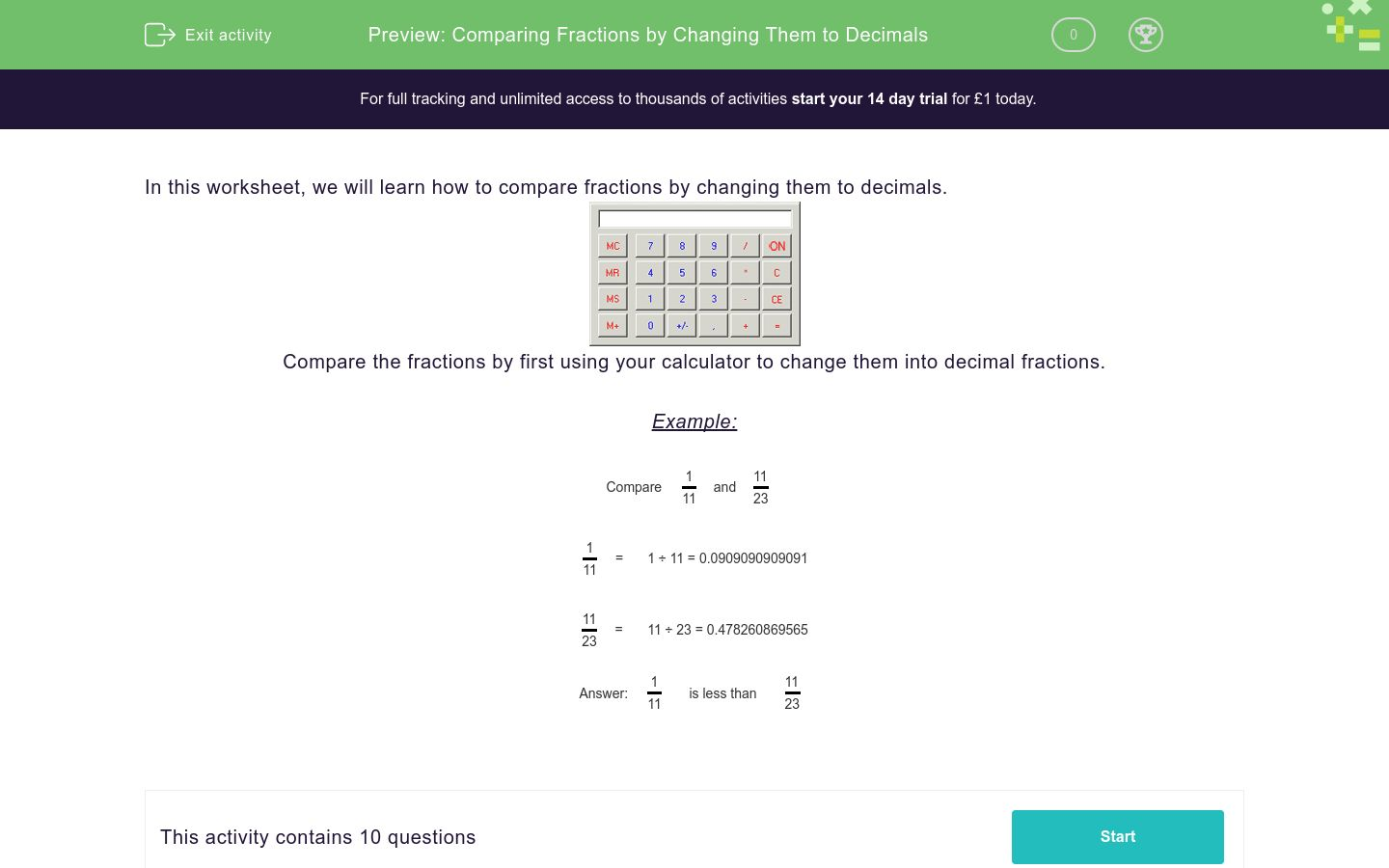# Comparing Fractions by Changing Them to Decimals

In this worksheet, students test the comparison of fractions by changing them to decimals.Key stage:  KS 3

Curriculum topic:   Number

Curriculum subtopic:   Use Calculators/Technology for Accuracy

Difficulty level:### QUESTION 1 of 10

In this worksheet, we will learn how to compare fractions by changing them to decimals.Compare the fractions by first using your calculator to change them into decimal fractions.

Example:

Compare
 1 11
and
 11 23

 1 11
=
1 ÷ 11 = 0.0909090909091

 11 23
=
11 ÷ 23 = 0.478260869565

 1 11
is less than
 11 23

Is the following comparision true or false

 8 10
>
 9 13

True

False

Is the following comparision true or false

 3 4
>
 6 7

True

False

Is the following comparision true or false

 1 24
<
 15 18

True

False

Is the following comparision true or false

 1 5
<
 2 6

True

False

Is the following comparision true or false

 6 9
>
 5 11

True

False

Is the following comparision true or false

 4 9
>
 5 11

True

False

Is the following comparision true or false

 14 20
<
 9 23

True

False

Is the following comparision true or false

 1 8
<
 13 15

True

False

Is the following comparision true or false

 7 13
<
 5 25

True

False

Is the following comparision true or false

 2 6
<
 6 7

True

False

• Question 1

Is the following comparision true or false

 8 10
>
 9 13

True
EDDIE SAYS
8 ÷ 10 = 0.8 9 ÷ 13 = 0.692
• Question 2

Is the following comparision true or false

 3 4
>
 6 7

False
EDDIE SAYS
3 ÷ 4 = 0.75 6 ÷ 7 = 0.857
• Question 3

Is the following comparision true or false

 1 24
<
 15 18

True
EDDIE SAYS
1 ÷ 24 = 0.0416 15 ÷ 18 = 0.833
• Question 4

Is the following comparision true or false

 1 5
<
 2 6

True
EDDIE SAYS
1 ÷ 5 = 0.2 2 ÷ 6 = 0.333
• Question 5

Is the following comparision true or false

 6 9
>
 5 11

True
EDDIE SAYS
6 ÷ 9 = 0.666 5 ÷ 11 = 0.454
• Question 6

Is the following comparision true or false

 4 9
>
 5 11

False
EDDIE SAYS
4 ÷ 9 = 0.444 5 ÷ 11 = 0.454
• Question 7

Is the following comparision true or false

 14 20
<
 9 23

False
EDDIE SAYS
14 ÷ 20 = 0.7 9 ÷ 23 = 0.391
• Question 8

Is the following comparision true or false

 1 8
<
 13 15

True
EDDIE SAYS
1 ÷ 8 = 0.125 13 ÷ 15 = 0.866
• Question 9

Is the following comparision true or false

 7 13
<
 5 25

False
EDDIE SAYS
7 ÷ 13 = 0.538 5 ÷ 25 = 0.2
• Question 10

Is the following comparision true or false

 2 6
<
 6 7

True
EDDIE SAYS
2 ÷ 6 = 0.333 6 ÷ 7 = 0.857
---- OR ----

Sign up for a £1 trial so you can track and measure your child's progress on this activity.

### What is EdPlace?

We're your National Curriculum aligned online education content provider helping each child succeed in English, maths and science from year 1 to GCSE. With an EdPlace account you’ll be able to track and measure progress, helping each child achieve their best. We build confidence and attainment by personalising each child’s learning at a level that suits them.

Get started